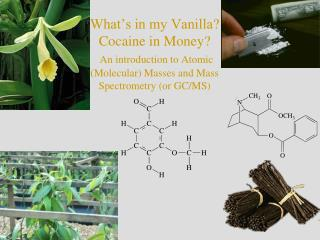DownloadDownload PresentationEvery atom has a certain number of protons, neutrons, and electrons.

# Every atom has a certain number of protons, neutrons, and electrons.

Télécharger la présentation## Every atom has a certain number of protons, neutrons, and electrons.

- - - - - - - - - - - - - - - - - - - - - - - - - - - E N D - - - - - - - - - - - - - - - - - - - - - - - - - - -
##### Presentation Transcript

1. What’s in my Vanilla?Cocaine in Money?An introduction to Atomic (Molecular) Masses and Mass Spectrometry (or GC/MS)

2. Every atom has a certain number of protons, neutrons, and electrons. • Z = atomic number (number of protons in an element, also determines which element you have, X) • X = abbreviation for the element • A = mass number (total number of protons and neutrons in a particular isotope of that element) • For a neutral atom, the number of electrons is equal to Z. If the atom is has a charge of +n, the number of electrons is equal to Z-n, if a charge of –n, the number of electrons is equal to Z+n

3. Complete the following table.

4. Isotopes of an element contain the same number of protons (i.e. the same element) but different numbers of neutrons • Examples: • Isotopes do not have to be radioactive • Many elements contain more than one naturally occurring isotope, a few have only one

5. Problem: if we know the number of protons and electrons in an atom, can we get the mass of an atom? • Possible solution: can we add up the masses of the individual nucleons? (mp) = 1.673 X 10-27 kg (me) = 9.11 X 10-31 kg (mn) = 1.675 X 10-27 kg Let’s try this for H and D

6. Problem: The masses of H and D are known to be: 1.674 X 10-27 kg and 3.344 X 10-27kg • We can’t add up the individual masses because when the nucleons combine to form atoms some of their mass is converted into energy to hold the nucleus together (binding energy) • What do we do?

7. Let’s choose a standard atomic mass unit (amu). Then we can measure the masses of all the atoms relative to that. • Definition: 1 atom of carbon-12 has a mass of exactly 12 amu or 1 amu is exactly ½ the mass of a carbon-12 atom. • Using this definition, 1 amu ( 1u) = 1.6605387 X 10-27 kg, H has a mass of 1.007825 amu , D has a mass of 2.0140 amu

8. Problem: Now that we’ve defined a standard, how do we measure masses of all the other atoms relative to that standard? • One solution: use a mass spectrometer that has been calibrated to the carbon-12 standard.

9. A mass spectrometer can measure mass to charge ratio of particles (single atoms or groups of atoms)

10. The mass spectrum is isotope specific

11. The atomic mass of an atom on the periodic table is really an average mass average relative atomic mass = A1p1 + A2p2 + … + AnPn An = exact mass of specific isotope pn = fractional abundance of isotope

12. Example Chlorine contains two naturally occurring isotopes: chlorine-35 (mass 34.968852 amu, 75.77% natural abundance) and chlorine-37 (mass 36.965903, 24.23% natural abundance). What is the average mass of chlorine?

13. OK, so what does this have to do with vanilla? • Natural vanilla is extracted from the vanilla orchid • Natural vanilla may contain more than one flavoring component • Sometimes natural vanilla can be adulterated with coumarin; this reduced costs, but is illegal because coumarin is hepatotoxic. • Artificial vanilla is produced from wood pulp • The chief constituent of both forms of vanilla is vanillin, C8H8O3

14. Problem: What is the molecular mass of a molecule of vanillin containing only 12C? one 13C?

15. OK, so what does this have to do with vanilla? • The metabolic pathway by which the vanilla orchid fixes carbon is different than that by which trees (used for wood pulp) do. This gives a slightly different 12Cto 13C ratio. • Using a high resolution mass spectrometer, we could measure the abundances of the 12C and 13C peak and determine the ratio to determine whether or not a sample is natural. • We probably won’t be able to make this distinction on our mass spectrometer, but...

16. Practice Sheet Objectives • Understand mass spectroscopy • Understand chromatography • Use GC/MS to examine differences between artificial vanilla extract, real vanilla extract, and vanilla beans • Identify minor flavoring components, preservatives, etc. • Identify coumarinas an illegal additive.

17. Gas Chromatography Mass Spectroscopy • Determine MM of a compound (mass of a molecule) • Determine structure of a compound • Determine ID of unknown • Used in conjunction • with GC to separate, • determine, and • quantify mixtures

18. A gas chromatograph will separate mixtures into components. This will lead to a chromatogram.

19. Each peak in a chromatogram has its own mass spectrum. mesitylene, C9H12 Other peaks are due to fragmentation of the molecule in the mass spec.

20. What Else Can We do With Mass Spectroscopy? • Arson analysis • Drug analysis • Trace analysis